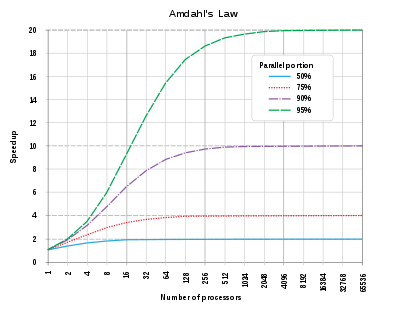# Amdhal’s Law

Speed up (P, N) = 1 / [ (1 — P) + P / N ]

N / [ 1 + α (N — 1) ]The theoretical speedup of the latency of the execution of a program (credit wikipedia).

# Brooks’s Law

`Number of communication channels = N x (N - 1) / 2`

# Universal Scalability Law

C(N) = N / [1 + α(N — 1) + βN (N — 1) ]

L = λ W

# Gustafson’s Law

S = s + p x N
S = (1 — s) x N
S = N + (1 — N) x s

# Metcalfe’s Law

Number of possible pair connections = N * (N — 1) / 2

Number of possible subgroups of a network = 2^NN − 1

--

--